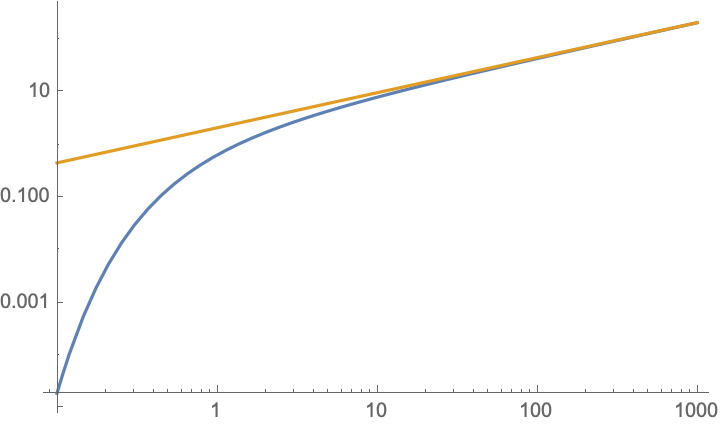# Definite integral of modified Bessel function of second kind

How do I integrate a modified Bessel function of the second kind as shown below? A good approximation of the definite integral is also ok, I do not need an exact solution.

$$\int_\frac{1}{\lambda}^{\infty}K_{5/3}(x) dx$$

$$\int_\frac{1}{\lambda}^{\infty}K_{5/3}(x) dx=2^{2/3} {\lambda}^{2/3} \Gamma \left(\tfrac{2}{3}\right) \, _1F_2\left(-\tfrac{1}{3};-\tfrac{2}{3},\tfrac{2}{3};\frac{1}{4 {\lambda}^2}\right)+\frac{27 \pi \sqrt{3} \, _1F_2\left(\frac{4}{3};\frac{7}{3},\frac{8}{3};\frac{1}{4 {\lambda}^2}\right)}{160\ 2^{2/3} {\lambda}^{8/3} \Gamma \left(-\frac{1}{3}\right)}-\frac{\pi }{\sqrt{3}}$$ The integral grows as $$2^{-1/3}\,3 \Gamma \left(5/3\right)\, \lambda^{2/3}$$ for large $$\lambda$$, see plot (blue is integral, gold is large-$$\lambda$$ asymptotics).